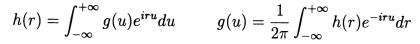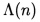## the Riemann-Weil explicit formula

The formula is most simply stated as[D. Hejhal, The Selberg Trace Formula for PSL(2,R) - Volume I, p. 35]

This is a generalisation of the Riemann-von Mangoldt explicit formula (which relates the zeros of the Riemann zeta function to the distribution of prime numbers). It also bears a striking and mysterious resemblance to the Selberg trace formula. Here h is a complex-valued function of a real variable satisfying certain conditions, and g and h are related by integral transforms:is the von Mangoldt function, defined to equal log p when n is a power of p, and to equal zero otherwise.

The formula first appeared, in a considerably more general form, in the following paper:

A. Weil, "Sur les 'formules explicites' de la théorie des nombres premiers", Comm. Lund (vol. dédié a Marcel Riesz) (1952) 252-265 [also available in volume 2 of his collected papers].

The formula given by Weil applies to a general algebraic number field K with a particular character called a Hecke grössencharacter. Associated with this pair is a Hecke L-function. The formula relates a sum over the nontrivial zeros of this L-function to a pair of sums: one over the powers of the finite prime elements of K, and one over the archimedean (infinite) prime places (or 'spots') of K.

If we take the simplest case where K is just the field of rationals, with trivial character, the associated Hecke L-function is just the Riemann zeta function, and the set of prime elements of K is just the familiar set of prime integers. The general formula then reduces to the one given by Hejhal above, where a sum over the nontrivial zeros of Riemann's zeta function is being related to a sum over the powers of primes.

Note that the sum in the rightmost term of Hejhal's formula, which involves the von Mangoldt function, can be rewritten in terms of a sum over all positive integral powers of all primes.

Section 2.6 of Z. Rudnick's "Zeta functions in arithmetic and their spectral statistics" contains a nice derivation of a variation on Weil's explicit formula.

Weil's formula is discussed further in

K. Barner's "On A. Weil's explicit formula", J. Reine und angew. math. 323 (1981) 139-152

Serge Lang, Algebraic Number Theory, Graduate Texts in Mathematics 110 (Springer-Verlag)

The versions of the formula given by Weil, Lang and Barner are all very similar, and clearly equivalent. They all involve a function F (satisfying certain conditions) which corresponds to the g in the version given by Hejhal above.

Lang points out that by choosing F(x) from the family of functions which vanishes when x < 0 or x > log y and is given by ex/2 when 0 < x < log y for some fixed y > 1, "one recovers a classical formula as in Ingham, Chapter IV". This chapter of Ingham's book contains a number of related explicit formulae of analytic number theory, including the Riemann-von Mangoldt explicit formula, so Lang is somewhat ambiguous in this statement.

A. Weil, "Sur les formules explicites de la théorie des nombres", Izv. Mat. Nauk (ser. Mat.) 36 (1972)

generalises the result to Artin-Hecke L-functions, and in

C.J. Moreno, "Explicit formulas in the theory of automorphic forms", Proc. Conference Rockefeller Univ. NY (1976) 73-216

the author presents analogous results for functions defined as Euler products associated to automorphic representations of GL2(A) where A is the adéle ring of the rationals.

D. Goldfeld, "Explicit Formulae as Trace Formulae", from Number Theory, Trace Formulas and Discrete Groups (K.E. Aubert, E. Bombieri and D. Goldfeld, eds.) (Academic, 1989) 281-288

"In his epoch-making paper, Selberg developed a general trace formula for discrete subgroups of GL(2,R). The analogies with the explicit formulae of Weil (relating very general sums over primes with corresponding sums over the critical zeroes of the zeta-function) are quite striking and have been the subject of much speculation over the years.

It is the object of this note to show that Weil's explicit formula can in fact be interpreted as a trace formula on a suitable space. The simplest space we have been able to construct for this purpose, at present, is the semidirect product of the ideles of norm one with the adeles, factored by the discrete subgroup Q* |X Q, the semidirect product of the multiplicative group of rational numbers with the additive group of rational numbers. We will show that for a suitable kernel function on this space, the conjugacy class side of the Selberg trace formula, is precisely the sum over the primes occurring in Weil's explicit formula.

This implies that the sum of the eigenvalues of the self-adjoint integral operator associated to the aforementioned kernel function is precisely the sum over the critical zeroes of the Riemann zeta-function occurring on the other side of Weil's formula. The relation between the eigenvalues of this integral operator and the zeroes of the zeta-function appears quite mysterious at present. What is lacking is a suitable generalization of the Selberg transform in this situation.

Finally, we should point out that our approach leads to various new equivalences to the Riemann Hypothesis, such as certain positivity hypotheses for the integral operators. Although we have worked over Q, for simplicity of exposition, it is not hard to generalize our relsults to L-functions of arbitrary number fields."

Explicit formulae generally are characterised as having the form of "the sum of a certain function extended to the prime powers [being] essentially equal to a sum of its Mellin transform extended to the zeros of the (relevant) zeta function."

"The paper of Riemann unveiled a deep relationship between the prime numbers and the zeros in the complex plane of a function already considered by Euler, the famous zeta function. A striking explicit formula was given by Riemann for the prime-counting function in terms of the zeros, and the generalizations obtained later all go by the name of 'Explicit Formulae'. This classical theory is surveyed in the books of Edwards and Ingham.

A particularly elegant formula was rigorously proven by von Mangoldt:

...

The literature on the Explicit Formulae is vast; it seems (to the author's knowledge) that Weil's version from 1952 subsumes most previously known such formulae. Its true merit probably lies in the adélic direction as will be explained later in this paper, although it is often advertised as having introduced a characteristic distribution-theoretic flavor to the subjects. It also introduced a funny psychological change in the understanding of what 'explicit' stands for. In the Riemann-von Mangoldt result one expresses an arithmetical counting function in an explicit manner in terms of the zeros. This is why the primes stand on the left and the zeros on the right of the equality sign. In Weil's tradition one expresses a sum over the zeros in an explicit manner in terms of the primes, hence the zeros are on the left and the primes on the right! Riemann wanted to say things about the primes from the zeros (and indeed this later led to a proof of the Prime Number Theorem), and Weil wanted to say things about the zeros from (some construction based on) the primes."

(from J.-F. Burnol, "The Explicit Formula in simple terms")

further commentary by Burnol on the relevance of the explicit formula

J.-F. Burnol, "The Explicit Formula and a propagator"

J.-F. Burnol, "The Explicit Formula and the conductor operator"

J.-F. Burnol, "On Fourier and zeta(s)"

fascinating speculative excerpt on the true nature of zeta

archive      tutorial      mystery      new      search      home      contact